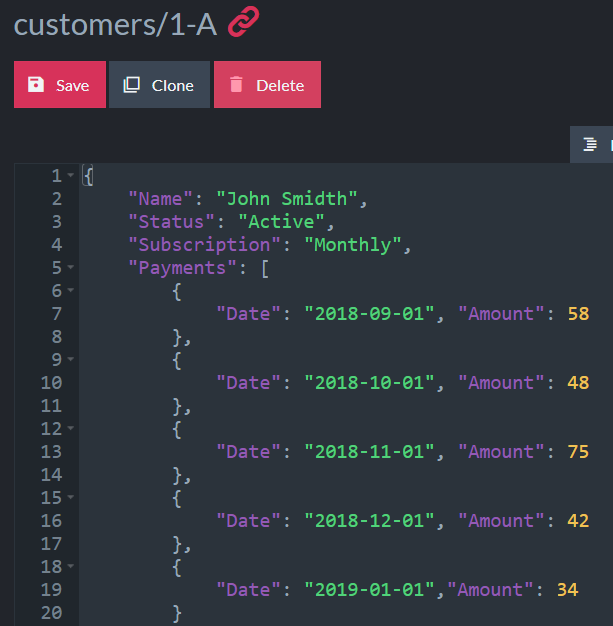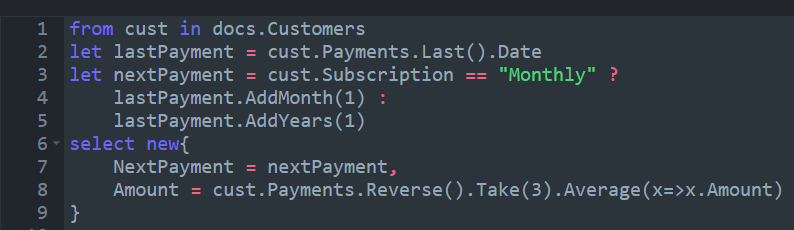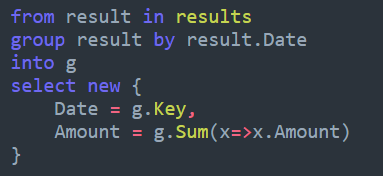## Data modeling with indexesPredicting the future

time to read 3 min | 505 words

Computation during indexes open up some nice  features when we are talking about data modeling and working with your data. In this post, I want to discuss predicting the future with it. Let’s see how we can do that, shall we?

Consider the following document, representing a (simplified) customer model:We have a customer that is making monthly payments. This is a pretty straightforward model, right?

We can do a lot with this kind of data. We can obviously compute the lifetime value of a customer, based on how much they paid us. We already did something very similar in a previous post, so that isn’t very interesting.

What is interesting is looking into the future. Let’s see how we can start simple, but figuring out what is the next charge rate for this customer. For now, the logic is about as simple as it can be. Monthly customers pay by month, basically. Here is the index:I’m using Linq instead of JS here because I’m dealing with dates and JS support for dates is… poor.

As you can see, we are simply looking at the last date and the subscription, figuring out how much we paid the last three times and use that as the expected next payment amount. That can allow us to do nice things, obviously. We can now do queries on the future. So finding out how many customers will (probably) pay us more than 100\$ on the 1st of Feb both easy and cheap.

We can actually take this further, though. Instead of using a simple index, we can use a map/reduce one. Here is what this looks like:And the reduce:This may seem a bit dense at first, so let’s de-cypher it, shall we?

We take the last payment date and compute the average of the last three payments, just as we did before. The fun part now is that we don’t compute just the single next payment, but the next three. We then output all the payments, both existing (that already happened) and projected (that will happen in the future) from the map function. The reduce function is a lot simpler, and simply sum up the amounts per month.

This allows us to effectively project data into the future, and this map reduce index can be used to calculate expected income. Note that this is aggregated across all customers, so we can get a pretty good picture of what is going to happen.

A real system would probably have some uncertainty factor, but that touches on business strategy more than modeling, so I don’t think we need to go into that here.